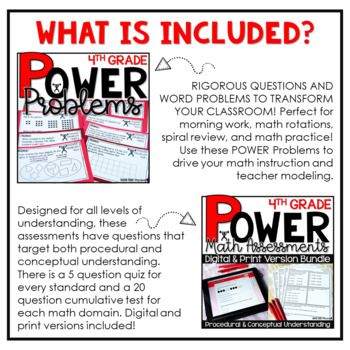# 4th Grade Math Word Problems Curriculum YEARLONG BUNDLE Print and Digital4th, Homeschool
Subjects
Standards
Resource Type
Formats Included
• Zip
•Google Apps™
Pages
500+
\$81.00
Bundle
List Price:
\$112.93
You Save:
\$31.93
\$81.00
Bundle
List Price:
\$112.93
You Save:
\$31.93This bundle contains one or more resources with Google apps (e.g. docs, slides, etc.).

### Description

The POWER Math Ultimate Bundle is everything you need for a successful year of math instruction! The resources found in this bundle were designed with the philosophy in mind that math should be POWERful. POWER stands for purposeful opportunities with engagement and rigor. You and your students deserve questions, assessments, printables and MORE that include questions that focus on conceptual learning.

***ALL RESOURCES INCLUDE A DIGITAL VERSION (GOOGLE SLIDES) AND PRINT VERSION!***

BUY THE BUNDLE AND SAVE!!

What is included:

POWER Problems- RIGOROUS QUESTIONS AND WORD PROBLEMS TO TRANSFORM YOUR CLASSROOM! Perfect for morning work, math rotations, spiral review, and math practice! Use these POWER Problems to drive your math instruction and teacher modeling.

POWER Math Assessments- Designed for all levels of understanding, these assessments have questions that target both procedural and conceptual understanding. There is a 5 question quiz for every standard and a 20 question cumulative test for each math domain. Digital and print versions included!

POWER Math Reference Mats- POWER Math Reference Mats are designed to assist teachers during math rotations. Housed with specific target skills for each level in your class, Guided Math Reference Mats come with various problems for you to give to your students when working in small groups. Group A is for students who are in need of remediation, Group B is for students working on grade level, and Group C is for students who need enrichment.

➥ POWER Math Journal- Designed for student practice, this journal has questions that target both procedural and conceptual understanding. You can bind the pages to make a journal or you can simply print out the pages for a print and go resource! 10 prompts/ questions are included per standard with space for work and answers. Most questions are short answer responses. You can use this math resource for test prep, math instruction, practice, or review!

➥ POWER Problems HD- Conceptual based questions to transform your homework assignments. Each sheet has four questions that are meaningful and rigorous. No more having your students complete repetitive procedural questions for homework that only skim the surface of thinking!

Standards & Topics Covered

Number and Operation in Base Ten

➥ 4.NB.1 - Place Value Concepts

➥ 4.NBT.2 - Number Form, Word Form, Expanded Form, and Comparing of whole numbers

➥ 4.NBT.3 - Rounding multi-digit whole numbers

➥ 4.NBT.4 - Adding & Subtracting Whole Numbers

➥ 4.NBT.5 - Multiplying whole numbers

➥ 4.NBT.6 - Dividing whole numbers

Operations & Algebraic Thinking

➥ 4.OA.1 - Interpreting multiplication equations

➥ 4.OA.2 - Multiplying and dividing word problems

➥ 4.OA.3 - Solving multistep word problems, including interpreting remainders

➥ 4.OA.4 - Factors and multiples, Identifying prime and composite numbers within 100

➥ 4.OA.5 - Patterns

Number and Operation - Fractions

➥ 4.NF.1 - Equivalent fractions

➥ 4.NF.2 - Comparing fractions

➥ 4.NF.3 - Adding and subtracting fractions with like denominators, decomposing fractions, adding and subtracting mixed numbers with like denominators

➥ 4.NF. 4 - Multiplying a fraction by a whole number

➥ 4.NF.5 - Adding and subtracting fractions with denominators of 10 and 100

➥ 4.NF.6 - Decimal notation for fractions with denominators of 10 and 100

➥ 4.NF.7 - Comparing decimals to hundredths

Measurement and Data

➥ 4.MD.1 - Measurement and converting measurement with the customary and metric systems of length, weight, mass, liquid volume, and time

➥ 4.MD.2 - Solving measurement word problems

➥ 4.MD.3 - Area and perimeter of rectangles

➥ 4.MD.4 - Line plots

➥ 4.MD.5 - Angles within a circle

➥ 4.MD.6 - Measuring angles with a protractor

➥ 4.MD.7 - Additive angle measurement, decomposing angles

Geometry

➥ 4.G.1 - Identifying points, lines, line segments, rays, angles, perpendicular and parallel lines in 2D shapes

➥ 4.G.2 - Classifying 2D figures, types of triangles

➥ 4.G.3 - Symmetry

Total Pages
500+
Included
Teaching Duration
Lifelong tool
Report this resource to TpT
Reported resources will be reviewed by our team. Report this resource to let us know if this resource violates TpT’s content guidelines.

### Standards

to see state-specific standards (only available in the US).
Interpret a multiplication equation as a comparison, e.g., interpret 35 = 5 × 7 as a statement that 35 is 5 times as many as 7 and 7 times as many as 5. Represent verbal statements of multiplicative comparisons as multiplication equations.
Multiply or divide to solve word problems involving multiplicative comparison, e.g., by using drawings and equations with a symbol for the unknown number to represent the problem, distinguishing multiplicative comparison from additive comparison.
Solve multistep word problems posed with whole numbers and having whole-number answers using the four operations, including problems in which remainders must be interpreted. Represent these problems using equations with a letter standing for the unknown quantity. Assess the reasonableness of answers using mental computation and estimation strategies including rounding.
Find all factor pairs for a whole number in the range 1-100. Recognize that a whole number is a multiple of each of its factors. Determine whether a given whole number in the range 1-100 is a multiple of a given one-digit number. Determine whether a given whole number in the range 1-100 is prime or composite.
Generate a number or shape pattern that follows a given rule. Identify apparent features of the pattern that were not explicit in the rule itself. For example, given the rule “Add 3” and the starting number 1, generate terms in the resulting sequence and observe that the terms appear to alternate between odd and even numbers. Explain informally why the numbers will continue to alternate in this way.

### Questions & Answers

Teachers Pay Teachers is an online marketplace where teachers buy and sell original educational materials.